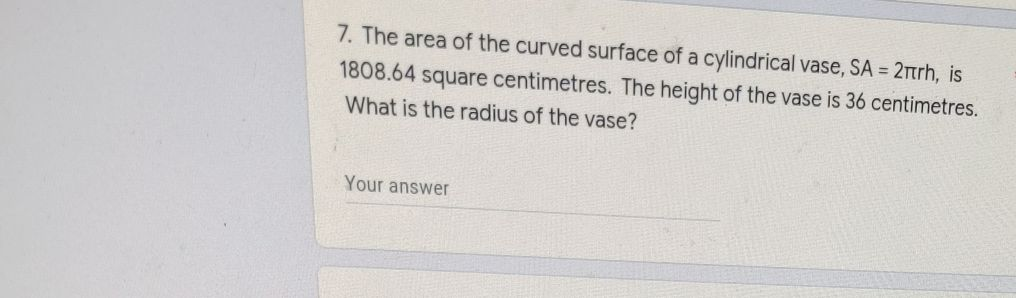### ¿Todavía tienes preguntas de matemáticas?

Pregunte a nuestros tutores expertos
Algebra
Pregunta7. The area of the curved surface of a cylindrical vase, $$S A = 2 \pi r h$$ , is

$$1808.64$$ square centimetres. The height of the vase is $$36$$ centimetres. What is the radius of the vase?